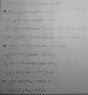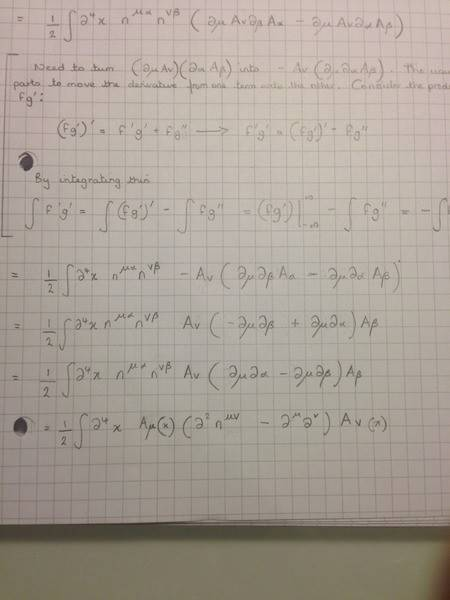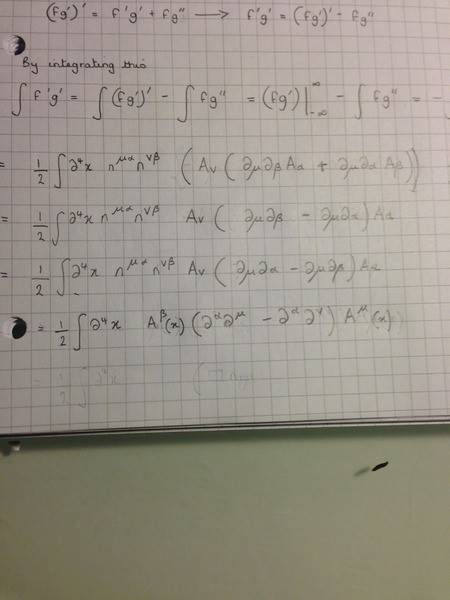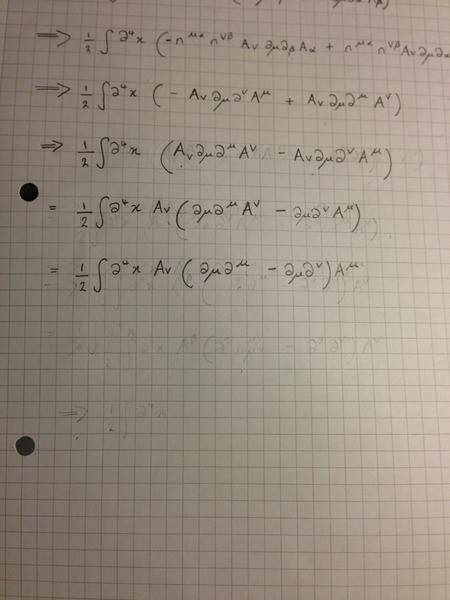# Electromagnetic field quantization

• smallgirl

#### smallgirl

1. Hey,
So I have to show this proof: $$\int d^{4}x(-\frac{1}{4}F_{\mu\nu}F^{\mu\nu})=\frac{1}{2}\int d^{4}xA^{\mu}(\square n_{\mu\nu}-\partial_{\mu}\partial_{\nu})A^{\nu}$$

2. Where
$$F_{\mu\nu}=\partial_{\mu}A_{\nu}-\partial_{\nu}A_{\mu}$$

3. ok, so I spent forever trying to type all of the above out, so you are going to have to have my solution as an image. I am not sure how right my solution is, and was wondering whether someone could have a look at it please? I'd be very grateful.

#### Attachments

•photo.jpg
30.2 KB · Views: 426
Last edited:
On the 8th line of the page, 7th line of the derivation, both factors of A appear to the left of the partials. Only one of them should be on the left, the other should be on the right of the partials. Also, there should be an overall minus sign. On the 9th line of the page, 8th line of the derivation, the factors of A are correctly placed, one on the left of the partials and one on the right.

My question to you is: Why are you allowed to move one of the factors of A to the left but not the other and why should there be an overall minus sign?

Also, on the 8th line of the derivation, you are very sloppy with the raising of indices and that needs to be fixed.

Hey,

I shall have a look in the morning with regards yours questions, however I'm not quite sure as to why there should be an overall minus sign? I took the minus sign from the -1/4 into the brackets and so lost the minus... I'm guessing I've gone wrong somewhere?

Explain what you did going from
$$\int d^4x\ \frac{1}{4} \eta^{\mu\alpha}\eta^{\nu\beta} ( -\partial_\mu A_\nu \partial_\alpha A_\beta+\partial_\mu A_\nu \partial_\beta A_\alpha-\partial_\nu A_\mu \partial_\alpha A_\beta+\partial_\nu A_\mu \partial_\beta A_\alpha)$$ to
$$\int d^4x\ \frac{1}{4} \eta^{\mu\alpha}\eta^{\nu\beta} [2 ( \partial_\mu A_\nu \partial_\beta A_\alpha-\partial_\mu A_\nu \partial_\alpha A_\beta)].$$ And what were you trying to do when you went from there to
$$\frac{1}{2} \int d^4x \ \eta^{\mu\alpha}\eta^{\nu\beta} A_\nu(x) A_\mu(x) ( \partial_\mu \partial_\beta - \partial_\mu \partial_\alpha)?$$

Explain what you did going from
$$\int d^4x\ \frac{1}{4} \eta^{\mu\alpha}\eta^{\nu\beta} ( -\partial_\mu A_\nu \partial_\alpha A_\beta+\partial_\mu A_\nu \partial_\beta A_\alpha-\partial_\nu A_\mu \partial_\alpha A_\beta+\partial_\nu A_\mu \partial_\beta A_\alpha)$$ to
$$\int d^4x\ \frac{1}{4} \eta^{\mu\alpha}\eta^{\nu\beta} [2 ( \partial_\mu A_\nu \partial_\beta A_\alpha-\partial_\mu A_\nu \partial_\alpha A_\beta)].$$ And what were you trying to do when you went from there to
$$\frac{1}{2} \int d^4x \ \eta^{\mu\alpha}\eta^{\nu\beta} A_\nu(x) A_\mu(x) ( \partial_\mu \partial_\beta - \partial_\mu \partial_\alpha)?$$

SO with the first line you have given I thought because everything is just dummy indices, and terms are repeated twice, I could just write it like that. I guess I have done something wrong?

With the second line you are writing about, I figured I could just take out terms common to both, but according to a previous replier, I can't write it like that...But I'm not sure as to why...

My question to you is: Why are you allowed to move one of the factors of A to the left but not the other and why should there be an overall minus sign?

So as I've said before I'm not quite sure as to why there should be an overall minus sign. I'm using Peskin and Schroeder as a guide and they don't have one in there either. Still trying to figure out the other thing you asked.

Anyways I made some changes, to try and correct the raising of indices.. not sure if what I have done is right...

#### Attachments

Last edited:
SO with the first line you have given I thought because everything is just dummy indices, and terms are repeated twice, I could just write it like that. I guess I have done something wrong?
Oh, okay. [strike]You have[/strike] I made a sign error in the first line I didn't notice earlier.

With the second line you are writing about, I figured I could just take out terms common to both, but according to a previous replier, I can't write it like that...But I'm not sure as to why...
Well, what you basically did there was akin to writing
$$\frac{df}{dx}\frac{dg}{dy} = fg \frac{d}{dx}\frac{d}{dy}.$$ Hopefully, you can see that's totally wrong.

The usual technique is to integrate by parts to move the derivative from one term onto the other. Integrating by parts is essentially using the product rule. Consider the product rule applied to differentiate fg':
$$(fg')' = f'g' + fg'' \hspace{1em} \rightarrow \hspace{1em} f'g' = (fg')' - fg''.$$ If you integrate this, you'd have
$$\int f'g' = \int (fg')' - \int fg'' = (fg')\big|_{-\infty}^\infty -\int fg'' = -\int fg''.$$ The first term, the integral of (fg')', is assumed to vanish, so the upshot is is that you can move the differentiation from one term onto the other at the cost of a minus sign.

Last edited:
I'm rather lost, as I didn't realize I needed to do integration by parts...I'm assuming then that the method for acquiring the factor of 2 wasn't incorrect then?..

Also I think I've still screwed up the raising and lowering of indices

The factor of 2 is fine. I confused myself with a sign error I made.

I'd take this one step at a time. Try to understand how to turn ##(\partial_\mu A_\nu)(\partial_\alpha A_\beta)## into ##-A_\nu (\partial_\mu \partial_\alpha A_\beta)##. Once you have that down, then worry about the indices.

So everything is fine up to the 5th line of the derivation? Sorry, I get confused easily, so trying to just make sure I'm ok up to a certain point...

I don't know how to delete this message. vela has said it all.

Last edited:
So I should keep the negative in front of the 1/4 for the moment then? I'm assuming I lose the negative sign later then...

So everything is fine up to the 5th line of the derivation? Sorry, I get confused easily, so trying to just make sure I'm ok up to a certain point...
It looks good right up to the line
$$\frac{1}{2} \int d^4x \ \eta^{\mu\alpha}\eta^{\nu\beta} A_\nu(x) A_\mu(x) ( \partial_\mu \partial_\beta - \partial_\mu \partial_\alpha).$$ That line doesn't make sense.

OK< shall have a bash from there then...

Try to understand how to turn ##(\partial_\mu A_\nu)(\partial_\alpha A_\beta)## into ##-A_\nu (\partial_\mu \partial_\alpha A_\beta)##. Once you have that down, then worry about the indices.

Hmm I think I get it now... So I can move one of the A's to the left of the brackets from both terms because of the integration by parts, but the second A term in both terms has to go on the right as it has not been taken out through integration by parts?

Last edited:
Right. In the original expression, the A's are both being acted on by operators, the ##\partial##'s, and the results are multiplied together. Integration by parts let's you move one of the operators from one factor onto the other, but in doing so, you pick up the minus sign. So in this particular case, you end up with ##A_\nu## multiplied by the result of applying ##\partial_\mu \partial_\alpha## to ##A_\beta##.

Hey,

So I now to sort out my indices... I think I might have it now?You can't pull the ##A_\beta## out of the parentheses like you did. In the first term, it was ##A_\alpha##, not ##A_\beta##.

So if I switch it to A$_{\alpha}$, and then relabel all the dummy indices at the end...should that be fine?

No, it sounds like you'd just be swapping ##A_\alpha## for ##A_\beta##.

So what should I do? I'm rather lost...

Any help would be appreciated...

I'd start all over and just simplify your task first by arguing, why
$$F^{\mu \nu} F_{\mu \nu} = F^{\mu \nu}(\partial_{\mu} A_{\nu}-\partial_{\nu} A_{\mu} ) = 2 F^{\mu \nu} \partial_{\mu} A_{\nu}.$$
Then write this as a total four-divergence and another term
$$2 F^{\mu \nu} \partial_{\mu} A_{\nu}=\partial_{\mu} [?]+[?].$$
Finally you can use that an integral over all four-space over a total four-divergence vanishes due to Gauss's theorem in four dimensions and the fact that we assume that the fields vanish rapidly enough at infinity.

This should help to derive the equation in the problem statement.

I can see how the first statement would work out due to -$F^{\mu\nu}$ being equal to $F^{\mu\nu}$

But I think I'd rather follow the method I've already started with...

Right. In the original expression, the A's are both being acted on by operators, the ##\partial##'s, and the results are multiplied together. Integration by parts let's you move one of the operators from one factor onto the other, but in doing so, you pick up the minus sign. So in this particular case, you end up with ##A_\nu## multiplied by the result of applying ##\partial_\mu \partial_\alpha## to ##A_\beta##.

Hey,

So I figure that integration by parts needs to be done twice which results in my attached picture, so that the A(alpha) can be taken out too, see attachment... Now not sure what to doThe following line can't be right.
$$\int d^4x\,\eta^{\mu\alpha}\eta^{\nu\beta}A_\nu(\partial_\mu\partial_\beta A_\alpha - \partial_\mu\partial_\alpha) A_\alpha.$$ If you multiply it out, the second term would be
$$\eta^{\mu\alpha}\eta^{\nu\beta}A_\nu\partial_\mu\partial_\alpha A_\alpha.$$ There are three ##\alpha##'s. When you see that, you should know something went wrong.

Go back to when you had
$$\int d^4x\,\eta^{\mu\alpha}\eta^{\nu\beta} A_\nu(-\partial_\mu\partial_\beta A_\alpha + \partial_\mu\partial_\alpha A_\beta).$$ Multiplying it out, you get two terms:
\begin{align*}
&\eta^{\mu\alpha}\eta^{\nu\beta} A_\nu \partial_\mu \partial_\beta A_\alpha \\
&\eta^{\mu\alpha}\eta^{\nu\beta} A_\nu \partial_\mu\partial_\alpha A_\beta
\end{align*} What do you end up with when you do the contractions to get rid of ##\alpha## and ##\beta##?

I get this:

$$\frac{1}{2}\int\partial^{4}x(-A_{\nu}\partial_{\mu}\partial^{\nu}A^{\mu}+A_{\nu}\partial_{\mu}\partial^{\mu}A^{\nu}$$

Last edited:
Good. Keep going.

Which after some rearrangement gives me...

$$\frac{1}{2}\int\partial^{4}xA_{\nu}(\partial_{\mu}\partial^{\mu}-\partial_{\mu}\partial^{\nu})A^{\mu}$$

So...what now?

Note: It should be ##d^4x##, not ##\partial^4x##.

Your first term is ##A_\nu \partial_\mu \partial^\mu A^\mu##. Again, you have an index showing up three times. I don't understand what you're doing to get that.

This:That doesn't really explain what you're thinking. What you're doing is like taking x(ay+bx) and saying it's equal to x(a+b)y. You're just replacing one variable by another for no apparent reason.

Hmm... I figured I could rearrange and use the results of integration by parts to achieve the rearrangement that I did.. But I guess what I did was wrong...

I'm thinking I might need to permute the indices and do some more raising and lowering?

$$\frac{1}{2}\int\partial^{4}x(-A_{v}\partial_{\mu}\partial^{\nu}A^{\mu}+A_{\nu}\partial_{\mu}\partial^{\mu}A^{\nu})$$

$$\frac{1}{2}\int\partial^{4}x(A_{v}\partial_{\mu}\partial^{\mu}A^{\nu}-A_{\nu}\partial_{\mu}\partial^{\nu}A^{\mu})$$

$$\frac{1}{2}\int\partial^{4}xA_{\nu}(\partial_{\mu}\partial^{\mu}A^{\nu}-\partial_{\mu}\partial^{\nu}A^{\mu})$$

$$\frac{1}{2}\int\partial^{4}xA_{\nu}(\square n_{\mu\nu}-\partial_{\mu}\partial^{\nu})A^{\mu}$$

Last edited:
Do you understand why what you did was wrong? Do you see that ##A_\nu \partial_\mu \partial^\mu A^\nu## and ##A_\nu \partial_\mu \partial^\mu A^\mu## aren't equal to each other? You've now made this same mistake several times, so it seems like you're just pushing symbols around without any understanding.